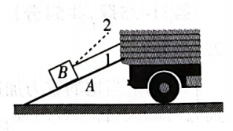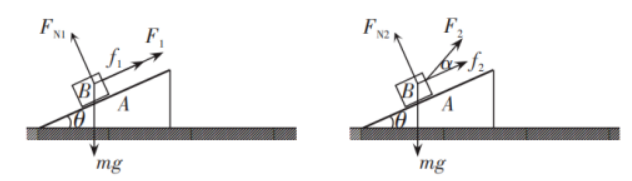$\text{A.}$ 第二次绳拉力的值可能小于第一次绳拉力的值 $\text{B.}$ 第二次 $B$ 受到摩擦力的值小于第一次 $A$ 受到摩擦力的值 $\text{C.}$ 第二次 $B$ 对 $A$ 作用力的值小于第一次 $B$ 对 $A$ 作用力的值 $\text{D.}$ 前、后两次 $B$ 对 $A$ 作用力的值大小不变

BC

#### 解析：①点击 收藏 此题， 扫码注册关注公众号接收信息推送（一月四份试卷,中1+高2+研1）
② 程序开发、服务器资源都需要大量的钱，如果你感觉本站好或者受到到帮助，欢迎赞助本站,赞助方式：微信/支付宝转账到 18155261033

 ①此题难易度如何 ②此题推荐度如何 确定